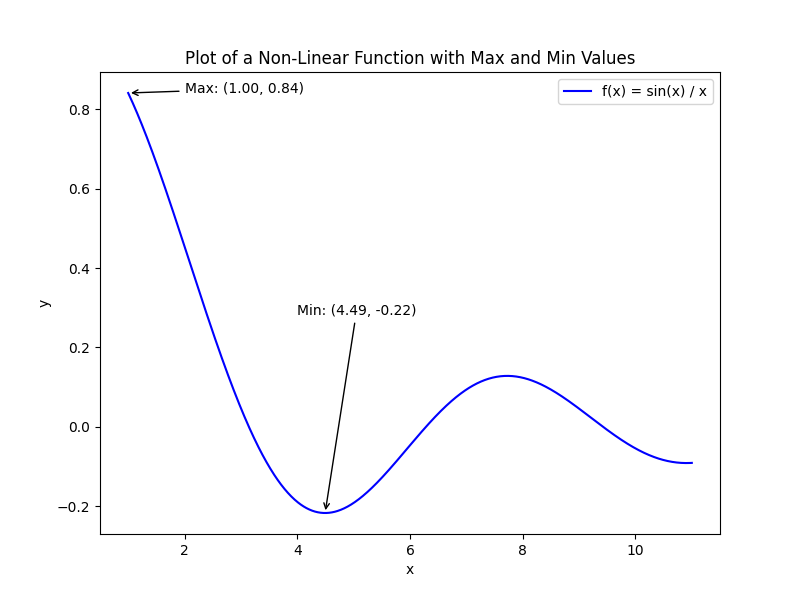Course Content

Mathematics for Data Analysis and Modeling

##Optimization Problem

In mathematics, an optimization problem is a type of mathematical problem where the objective is to find the best solution among all possible feasible solutions. The goal is to optimize (maximize or minimize) a certain objective function, subject to a set of constraints.
The solution to an optimization problem is a set of values for the decision variables that satisfy the constraints and optimize the objective function. Depending on the problem and constraints, the solution can be unique or may have multiple optimal solutions.
The most simple optimisation problem can be defined as follows:This task can be formulated as follows: we have to find the value from the domain `X` (the point of maximum/minimum) for which the function `f` will have a maximum (minimum) value in codomain `Y`.### How can we solve the optimization problem?

There is no one-size-fits-all approach to solving optimization problems, as the approach often depends on the type of function and the domain in which we seek the optimal solution. However, when dealing with a function of one variable, there is a specific algorithm that can effectively solve the optimization problem:The solutions of the equation described in the first point is also called critical value of the function `f`.
Now let's look how to solve the optimization problem in Python:We used `sp.solve(f, x)` method in the code above. This method finds a numerical solution of the equation `f(x) = 0` with respect to variable `x`.

Everything was clear?

Section 3. Chapter 4